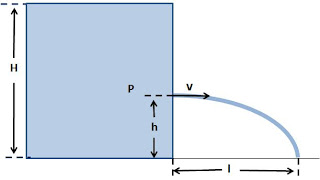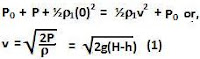## Thursday, May 7, 2009

### Irodov Problem 1.319Suppose that the hole is made at a height h. The fluid pressure P at this height is given by. Let the atmospheric pressure be Po.The water just to the left of the hole is assumed to be still, in other words it has no velocity.

Just to the right of the hole, say the water shoots out with a horizontal velocity v. The water coming out of the hole to enter a zone with pressure equal to atmospheric pressure and so from Bernoulli's equation we have,Gravity pulls the water down with an acceleration of g, and so the time t taken by water to reach the surface from a height h is given byThe horizontal distance traveled by the water before its hits the ground is given by,The extrema (maxima/minima) of l lies at,Also at this value of h, it can be verified that the second derivative is negative indicating a maxima.

The distance traveled by the water for this value of h is given by substituting h = H/2 in l and is given by H.